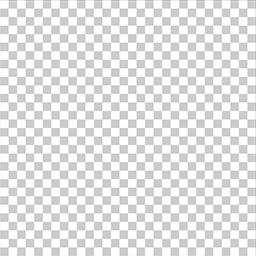# Animating Hulu Pre-Roll with a WebGL Fragment Shader

last updated on 2020-02-27

In this tutorial you'll learn how to create video-like animations, using WebGL fragment shaders and HTML canvas.

This is the second part of a series of 4 articles - if you jut landed here you may want to start `there instead`

The Hulu example is great to begin with as it only contains a single color and its shapes are not too complex to be replicated in a shader.

#### Define primitives

The first step is to understand how to draw primitive shapes. By superposing, masking and composing them together we can create more complex shapes.

For this example we only need to use two primitives:

• rectangles (could be square or not)
• rectangles with rounded corners

We first need to define them as functions:

``````// from https://thebookofshaders.com/07/
float square(in vec2 _st, in vec2 _size){
_size = vec2(0.5) - _size*0.5;
vec2 uv = smoothstep(_size, _size+vec2(0.001), _st);
uv *= smoothstep(_size, _size+vec2(0.001), vec2(1.0)-_st);
return uv.x*uv.y;
}

// from http://www.iquilezles.org/www/articles/distfunctions/distfunctions.htm
float roundBox(vec2 pos, vec2 bounds, float radius) {
return 1.0 - smoothstep(0.0, 0.005, length(max(abs(pos) - bounds, 0.0)) - radius);
}``````

These operations allows us to define whether the pixel is inside the shape or outside, returning 1.0 for inside and 0.0 for outside. The `smoothstep(min, max, val)` to define the a gradient surface versus defining sharp edge.

Let's look at how to use it next.

#### Draw a rectangle

To draw a rectangle we'll use the `square` method defined above. First get the value in a float, them multiply it to the color we want to apply.

For example to draw the letter `l` of Hulu, we just need to create a green rectangle with a higher `scale.y` and thin `scale.x`, so it s thin and tall.

``````vec3 drawLetterL (vec2 st) {
float box = square(st + vec2(0.0, -0.27), vec2(0.16, 0.75));
vec3 color = vec3(box);
return color;
}``````

Ok that was simple one, next we look into how to combine multilpe shapes to create more complex ones.

#### Draw more complex letters

Now let's step it up a bit and look into subtracting shapes, a technique also referred to as `masking`.

In order to draw a `U` we follow these steps:

• draw a rectangle with rounded corners,
• draw a smaller one in the middle and subtract it from the initial one,
• crop the top by subtracting a wide rectangle.In GLSL it looks like that:

``````vec3 drawLetterU (vec2 st) {
vec3 color = vec3(0.0);

// main base
float box = roundBox(st - vec2(0.5, 0.75), vec2(0.125, 0.2), 0.15);
color = vec3(box);

box = roundBox(st - vec2(0.5, 0.74), vec2(0.05, 0.12), 0.05);
color = mix(color, vec3(0), box);

// crop the top
box = square(st + vec2(0.0, -0.55), vec2(0.6, 0.4));
color = mix(color, vec3(0), box);

return color;
}``````

Notice how when blending layers it's common to use `mix(value1, value2, control)`.

So we know how to make a `l` and just looked at how to make a `u`. Can you guess how we could further combine these shapes to create the missing `h` letter?

From there it's up to your imagination, see how far modern heroes are taking it

### Animation Timing

If you've read `part 1 of this series` you may recall our shaders are setup with a uniform of type float `u_time` binded with the slider value with a range of `0` to `10`.

We use this `u_time` value to control our animation. In order to get there we need a way to map the current time to a specific desired value.

For example we want to be able to write a command for setting the alpha from 0 to 1, starting at 3 seconds and fully visible at 4 second in the timeline.

``````float mapTime (float from, float to, float time) {
return clamp((time - from) / (to - from), 0.0, 1.0);
}``````

With the function and the use case described above, it would translate to something like:

``float alpha = mapTime(3.0, 4.0, u_time);``

With these basic tools in hand, we can start thinking more creatively combining shapes and time.

So that's the basics, in the `next article` we'll dig into the same topics with a bit more depth.

Hope you enjoyed this tutorial! The stuff I write about is a way for me to improve my learnings - probably just like you right now. So please if you catch any issue or want to suggest an edit, use the section below or reach out on twitter. Cheers!